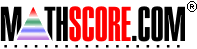Math Practice Online > free > lessons > Florida > 6th grade > Decimal Rounding

## Decimal Rounding

Practice rounding decimals up to the billionth

 Sample Problems for Decimal Rounding Lesson for Decimal Rounding

### This topic aligns to the following state standards

Grade 6: Num 1. Knows an appropriate estimation technique for a given situation using whole numbers (for example, clustering, compatible number, front-end).
Grade 7: Num 1. Knows an appropriate estimation technique for a given situation using whole numbers, fractions and decimals.
Grade 8: Num 1. Knows appropriate estimation techniques for a given situation using real numbers.

Copyright Accurate Learning Systems Corporation 2008.